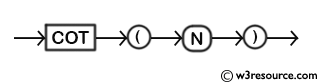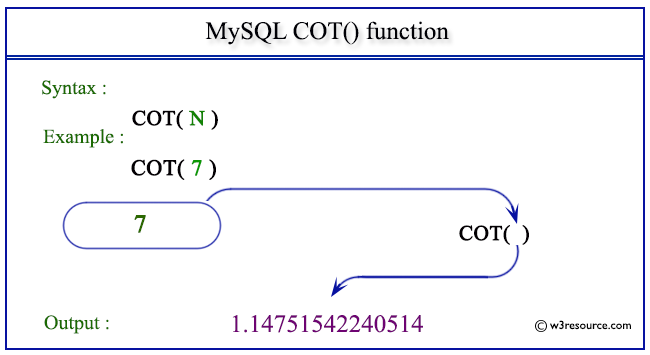# MySQL COT() function

## COT() function

MySQL COT() returns the cotangent of a number.

This function is useful in -

• COT() is a fundamental trigonometric function used in mathematics, physics, engineering, and various scientific fields.
• This function finds the angle at which the tangent is equal to a given value by taking the reciprocal of the tangent.
• COT() is employed in the design and analysis of antennas, as well as in the study of wave propagation in various mediums.
• The COT() function represents oscillatory behavior and is used to solve differential equations with trigonometric functions in simulations and modeling.
• In electrical engineering, COT() is used in the analysis of alternating current (AC) circuits, especially in scenarios involving reactive components like inductors and capacitors.
• In computer graphics and animation, COT() can be used to create various effects, particularly those involving oscillatory motion or wave-like behavior.
• In the study of earthquakes and vibrations, COT() is employed to model the oscillatory behavior of structures and the propagation of seismic waves.

Syntax:

```COT(N);
```

Argument:

Name Description
N A number whose cotangent value is to be retrieved.

Syntax Diagram:MySQL Version: 8.0

Pictorial presentation of MySQL COT() functionExample of MySQL COT() function

Code:

``````SELECT COT(7);
```
```

Explanation:

The above MySQL statement will return the cotangent of the number specified as an argument.

Output:

```mysql> SELECT COT(7);
+------------------+
| COT(7)           |
+------------------+
| 1.14751542240514 |
+------------------+
1 row in set (0.01 sec)
```

All Mathematical Functions

Previous:COS()
Next: CRC32()

﻿